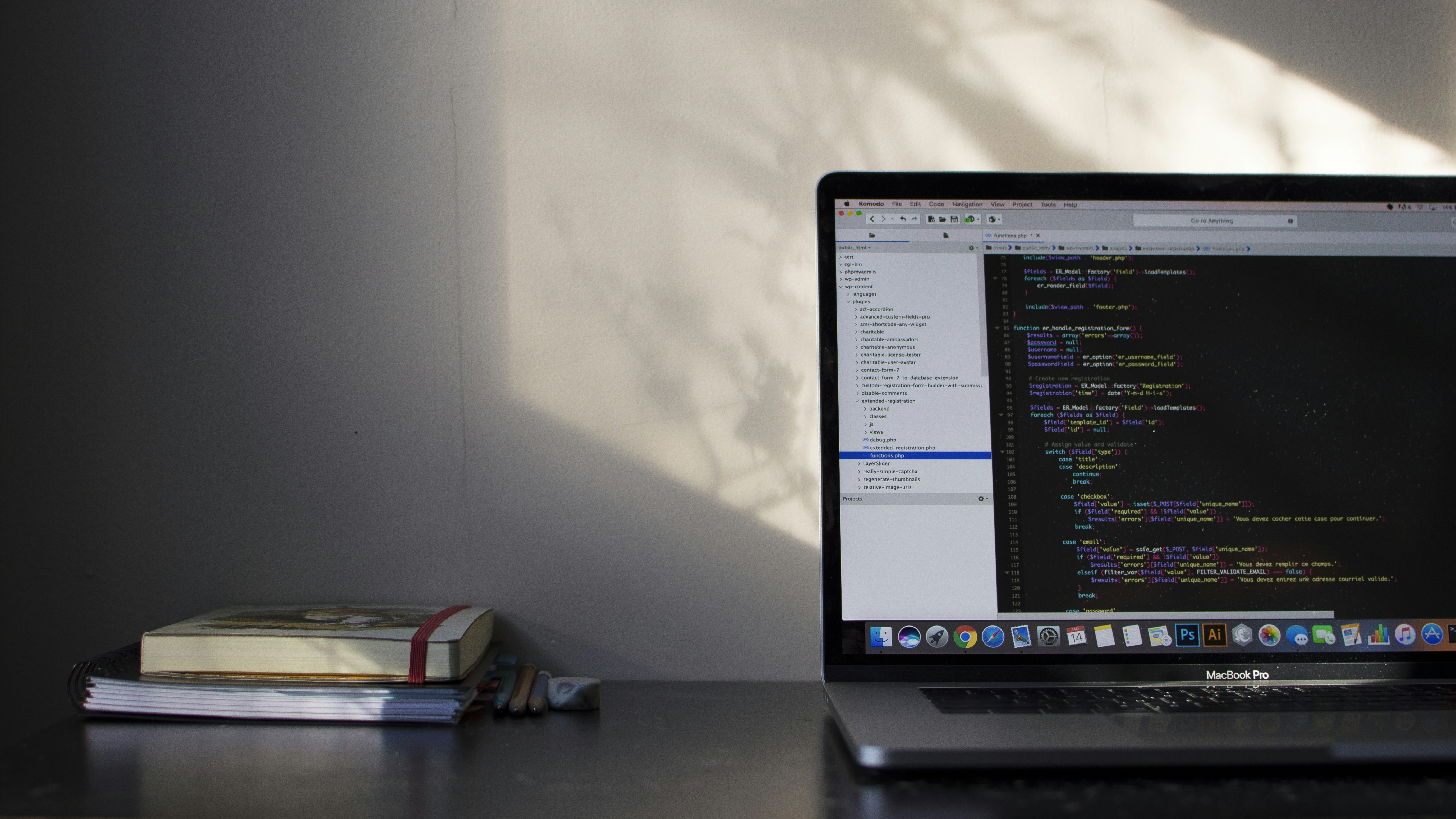Home » Solve All Algorithm Problems – using Two Pointers technique

# Solve All Algorithm Problems – using Two Pointers techniqueTwo pointers is a common coding technique to solve some algorithm problems, not all, but many algorithm problems can be solved by using it.

To be more professional on algorithm and data structure, LeetCode does help, however, the problem is that there are two many interview coding questions there, it you want to finish all of them, it might take you a very long time.

Instead of going through all of them, it is better to know how to solve it and what coding techniques can be used to solve it and what is the performance (Big O Time/Space) by using the technique.

In this article, we would like to discuss about 2 pointers technique. (Please be noticed that the pointer here does not only mean a pointer like in C/C++, but it also stands for the index of an array.)

## Keywords

• Sorted Array
• Or it is OK to Sort the Array Firstly.

This is basically the usage cases for the two pointers.

### Use Cases

• Remove Duplicated From a Sorted Array
• Find Pair Sum
• Closest Pair Sum
• Find a triplet (3 Elements Sum to 0)
• Or Find even more elements
• And more related to sorted array

## Example – Remove Duplication from LeetCode

We do not have the right to copy the content from LeetCode, but please do click the link above and go through the question first, it is basically to remove duplicated items from an sorted array.

### 1. Simple Python Solution

``````class Solution:
def removeDuplicates(self, nums: List[int]) -> int:
return len(set(nums))
``````

This solution will not be accepted, because the requirement does have extra requirements.

• Do update the array directly, we generate a new set here, not updated the original list
• As for Space Complexity, it is required to be O(1), but in the solution above, it is O(n)coding in React/ 2 pointers/ Simple Algorithm

### 2. Two Pointers Solution Python

Runtime: 76 ms, faster than 98.04% of Python3 online submissions for Remove Duplicates from Sorted Array.

Memory Usage: 15.5 MB, less than 72.67% of Python3 online submissions for Remove Duplicates from Sorted Array.

The 2 Pointers Solution Performance
``````class Solution:
def removeDuplicates(self, nums: List[int]) -> int:
l = len(nums)
if l == 0:
return 0
else:
i = 0
for j in range (1, l):
if (nums[j] != nums[i] ):
i +=1
nums[i] = nums[j]
return i+1

``````

As you can see above, there are 2 pointers here, j is the fast one and i is the slow one, some times 2 pointers are not like this fast and slow, but one is from start and the other is from the end, we will talk about it in the future.

• Time O(n), only one for-loop
• Space O(1), maximum one item is changed during the loop
• Besides, there are more solutions here.

## 2 thoughts on “Solve All Algorithm Problems – using Two Pointers technique”

1.Amazing webpage thank you for making it!